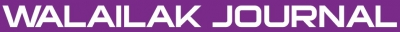A Haar Wavelet Study on Convective-Radiative Fin under Continuous Motion with Temperature-Dependent Thermal Conductivity

Adivi Sri Venkata RAVI KANTH, Niyan Uday KUMAR

Abstract

In this paper, the Haar wavelet method is applied to find an approximate solution for heat transfer in moving fins with temperature-dependent thermal conductivity losing heat through both convection and radiation to the surroundings. The effects of various significant parameters involved in the problem, such as the thermal conductivity parameter a, sink temperature , convection-radiation parameter Nc, radiation-conduction parameter Nr, and Peclet number Pe on the temperature profile of the fin, is discussed and physical interpreted through illustrative graphs.

doi:10.14456/WJST.2014.40

Keywords

Haar wavelets, extended surface, convective-radiative heat transfer, temperature-dependent thermal conductivity, Newton’s method

PDF

References

DQ Kern and AD Kraus. Extended Surface Heat Transfer. McGraw-Hill, New York, 1972.

AD Kraus, A Aziz and J Welty. Extended Surface Heat Transfer. John Wiley and Sons, New York, 2001.

JH Lienhard IV and JH Lienhard V. A Heat Transfer Textbook. Phlogiston Press, Cambridge, Massachusetts, 2003.

CH Chiu and CK Chen. A decomposition method for solving the convective longitudinal fins with variable thermal conductivity. Int. J. Heat Mass Tran. 2002; 45, 2067-75.

C Arslanturk. A decomposition method for fin efficiency of convective straight fins with temperature-dependent thermal conductivity. Int. Commun. Heat Mass Tran. 2005; 32, 831-41.

A Rajabi. Homotopy perturbation method for fin efficiency of convective straight fins with temperature-dependent thermal conductivity. Phys. Lett. A 2007; 364, 33-7.

G Domairry and M Fazeli. Homotopy analysis method to determine the fin efficiency of convective straight fins with temperature-dependent thermal conductivity. Commun. Nonlinear Sci. Numer. Simulat. 2009; 14, 489-99.

AA Joneidi, DD Ganji and M Babelahi. Differential transformation method to determine fin efficiency of convective straight fins with temperature dependent thermal conductivity. Int. Commun. Heat Mass Tran. 2009; 36, 757-62.

DB Kulkarni and MM Joglekar. Residue minimization technique to analyze the efficiency of convective straight fins having temperature-dependent thermal conductivity. Appl. Math. Comput. 2009; 215, 2184-91.

R Das. A simplex search method for a conductive-convective fin with variable conductivity. Int. J. Heat Mass Tran. 2011; 54, 5001-9.

A Aziz and JY Benzies Application of perturbation techniques to heat-transfer problems with variable thermal properties. Int. J. Heat Mass Tran. 1976; 19, 271-6.

H Nguyen and A Aziz. Heat transfer from convecting-radiating fins of different profile shapes. Warme und Stoffubertragung 1992; 27, 67-72.

LT Yu and CK Chen. Application of Taylor transformation to optimize rectangular fins with variable thermal parameters. Appl. Math. Model. 1998; 22, 11-21.

MN Bouaziz and A Aziz. Simple and accurate solution for convective-radiative fin with temperature dependent thermal conductivity using double optimal linearization. Energ. Convers. Manage. 2010; 51, 2276-782.

A Aziz and F Khani. Convection-radiation from a continuous moving fin of variable thermal conductivity. J. Franklin Inst. 2011; 348, 640-51.

A Aziz and RJ Lopez. Convection-radiation from a continuously moving, variable thermal conductivity sheet or rod undergoing thermal processing. Int. J. Therm. Sci. 2011; 50, 1523-31.

M Torabi, H Yaghoobi and A Aziz. Analytical solution for convective-radiative continuously moving fin with temperature-dependent thermal conductivity. Int. J. Thermophys. 2012; 33, 924-41.

CF Chen and CH Hsiao. Haar wavelet method for solving lumped and distributed-parameter systems. IEEE Proc. Control Theory Appl. 1997; 144, 87-94.

CH Hsiao and WJ Wang. Haar wavelet approach to nonlinear stiff systems. Math. Comput. Simulat. 2001; 57, 347-53.

CH Hsiao. Haar wavelet approach to linear stiff systems. Math. Comput. Simulat. 2004; 64, 561-7.

K Maleknejad, F Mirzaee and S Abbasbandy. Solving linear integro-differential equations system by using rationalized Haar functions method. Appl. Math. Comput. 2004; 155, 317-28.

U Lepik. Numerical solution of differential equations using Haar wavelets. Math. Comput. Simulat. 2005; 68, 127-43.

U Lepik. Haar wavelet method for nonlinear integro-differential equations. Appl. Math. Comput. 2006; 176, 324-33.

R Kalpana and SR Balachandar. Haar wavelet method for the analysis of transistor circuits. Int. J. Electron. Commun. 2007; 61, 589-94.

E Babolian and A Shahsavaran. Numerical solution of nonlinear Fredholm integral equations of the second kind using Haar wavelets. J. Comput. Appl. Math. 2009; 225, 87-95.

G Hariharan, K Kannan and KR Sharma. Haar wavelet method for solving Fisher’s equation. Appl. Math. Comput. 2009; 211, 284-92.

S Ul-Islam, I Aziz and B Sarler. The numerical solution of second-order boundary-value problems by collocation method with the Haar wavelets. Math. Comput. Model. 2010; 52, 1577-90.

S Zhi and C Yong-yan. A spectral collocation method based on Haar wavelets for Poisson equations and biharmonic equations. Math. Comput. Model. 2011; 54, 2858-68.

S Zhi and C Yong-yan. Application of Haar wavelet method to eigen value problems of high order differential equations. Appl. Math. Model. 2012; 36, 4020-6.

S Zhi and C Yong-yan. Solving 2D and 3D Poisson equations and biharmonic equations by the Haar wavelet method. Appl. Math. Model. 2012; 36, 5143-61.

Refbacks

• There are currently no refbacks.Online ISSN: 2228-835X

http://wjst.wu.ac.th

Last updated: 17 May 2019